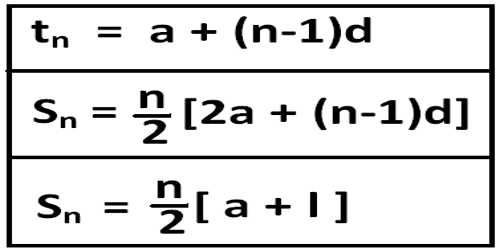Mathematic

# Arithmetic Progression FormulaA sequence of numbers is known as an arithmetic progression (A.P.) if the difference between the term and the preceding term is always same or constant. An arithmetic progression is a sequence of numbers such that the difference between any two successive members is constant.

For example,

• the sequence 1, 2, 3, 4, … is an arithmetic progression with common difference 1.
• Second example: the sequence 3, 5, 7, 9, 11,… is an arithmetic progression with common difference 2.
• the sequence 20, 10, 0, -10, -20, -30, … is an arithmetic progression with common difference -10.Arithmetic Progression Formula

1. Let ‘a’ be the first term and ‘d’ the common difference of an Arithmetic Progression. Then its

(i) General term (nth term) = tn = a + (n – 1)d

(ii) The sum of the first n terms = Sn = n/2(a + l) = [Number of terms / 2] (First term + Last term)

or, Sn = n/2 [2a + (n – 1)d] where l = last term = nth term = a + (n – 1)d.

1. (i) The sum of first n natural numbers (S2)= n/2(n + 1)

i.e., 1 + 2 + 3 + 4 + 5 + ……………….. + n = n/2 (n + 1)

(ii) The sum of the squares of first n natural numbers (Sn)= 1/6 n (n + 1)(2n + 1)

i.e., 12 + 22 + 32 + 42 + 52 + ………………. + n2  = 1/6 n (n + 1)(2n + 1)

(iii) The sum of the cubes of first n natural numbers (S2) = {1/2 n (n +1)}2

i.e., 13 + 23 + 33 + 43 + 53 + ……….. + n3 = {1/2 n (n +1)}2

1. The arithmetic mean between two given quantities ‘a’ and ‘b’ = ½ × (Sum of the given quantities) = a+b2a+b2.
2. (i) If the sum of three terms in Arithmetic Progression be given, assume the numbers as a – d, a and a + d. Here common difference is d.

(ii) If the sum of four terms in Arithmetic Progression be given, assume the numbers as a – 3d, a – d, a + d and a + 3d.

(iii) If the sum of five terms in Arithmetic Progression be given, assume the numbers as a – 2d, a – d, a, a + d and a + 2d. Here common difference is 2d.

(iv) If the sum of six terms in Arithmetic Progression be given, assume the numbers as a – 5d, a – 3d, a – d, a + d, a + 3d and a + 5d. Here common difference is 2d.

Information Source